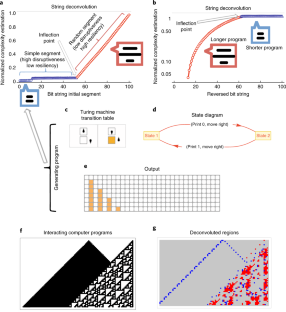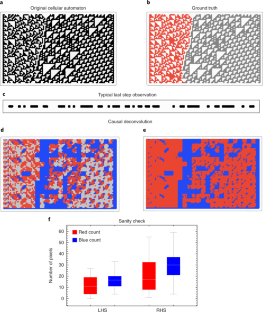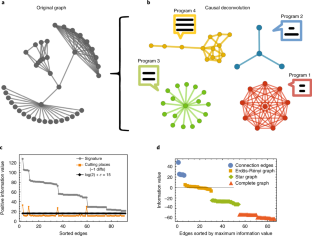Thank you for visiting nature.com. You are using a browser version with limited support for CSS. To obtain the best experience, we recommend you use a more up to date browser (or turn off compatibility mode in Internet Explorer). In the meantime, to ensure continued support, we are displaying the site without styles and JavaScript.

# Causal deconvolution by algorithmic generative models

### Subjects

A preprint version of the article is available at arXiv.

## Abstract

Complex behaviour emerges from interactions between objects produced by different generating mechanisms. Yet to decode their causal origin(s) from observations remains one of the most fundamental challenges in science. Here we introduce a universal, unsupervised and parameter-free model-oriented approach, based on the seminal concept and the first principles of algorithmic probability, to decompose an observation into its most likely algorithmic generative models. Our approach uses a perturbation-based causal calculus to infer model representations. We demonstrate its ability to deconvolve interacting mechanisms regardless of whether the resultant objects are bit strings, space–time evolution diagrams, images or networks. Although this is mostly a conceptual contribution and an algorithmic framework, we also provide numerical evidence evaluating the ability of our methods to extract models from data produced by discrete dynamical systems such as cellular automata and complex networks. We think that these separating techniques can contribute to tackling the challenge of causation, thus complementing statistically oriented approaches.

## Access options

from\$8.99

All prices are NET prices.Fig. 1: Proof of concept applied to a binary string composed of two segments with different underlying generating mechanisms (computer programs).Fig. 2: Training-free separation of intertwined programs despite their statistical similarity from an observer’s perspective.Fig. 4: Unsupervised graph deconvolution identifies each different topological generating mechanism.

## Data availability

The data that support the plots within this paper are available from the corresponding author upon request.

## References

1. 1.

Zenil, H. et al. An algorithmic information calculus for causal discovery and reprogramming systems. Preprint at https://doi.org/10.2139/ssrn.3193409 (2018).

2. 2.

Zenil, H., Kiani, N. A., Zea, A. A., Rueda-Toicen, A. & Tegnér, J. Data dimension reduction and network sparsification based on minimal algorithmic information loss. Preprint at https://arxiv.org/abs/1802.05843 (2018).

3. 3.

Lloyd, S. P. Least squares quantization in PCM. IEEE Trans. Inform. Theory 28, 129–137 (1982).

4. 4.

Kaufman, L. & Rousseeuw, P. J. in Statistical Data Analysis Based on the L1-Norm and Related Methods (North-Holland, Amsterdam, 1987).

5. 5.

Ben-Hur, A., Horn, D., Siegelmann, H. & Vapnik, V. N. Support vector clustering. J. Mach. Learn. Res. 2, 125–137 (2001).

6. 6.

Milo, R. et al. Network motifs: simple building blocks of complex networks. Science 298, 824–827 (2002).

7. 7.

Newman, M. E. J. Finding community structure in networks using the eigenvectors of matrices. Phys. Rev. E 74, 036104 (2006).

8. 8.

Benczur, A. & Karger, D. R. Approximating s-t minimum cuts in O(n 2)-time. In Proc. Twenty-Eighth Annual ACM Symposium on the Theory of Computing 47–55 (ACM, 1996).

9. 9.

Spielman, D. A. & Srivastava, N. Graph sparsification by effective resistances. In Proc. Fortieth Annual ACM Symposium on Theory of Computing 563–568 (ACM, 2008).

10. 10.

Spielman, D. A. & Teng, S.-H. Spectral sparsification of graphs. SIAM J. Comput. 40, 981–1025 (2011).

11. 11.

Liu, M., Liu, B. & Wei, F. Graphs determined by their (signless) Laplacian spectra. Electron. J. Linear Algebra 22, 112–124 (2011).

12. 12.

Granger, C. W. J. Investigating causal relations by econometric models and cross-spectral methods. Econometrica 37, 424–438 (1969).

13. 13.

Schreiber, T. Measuring information transfer. Phys. Rev. Lett. 85, 461–464 (2000).

14. 14.

Pearl, J. Causality: Models, Reasoning and Inference (Cambridge University Press, Cambridge, 2000).

15. 15.

Solomonoff, R. J. A formal theory of inductive inference: parts 1 and 2. Inform. Control 7, 1–22–224–254 (1964).

16. 16.

Watanabe, S. in Frontiers of Pattern Recognition (ed. Watanabe, S.) 561–568 (Academic Press, New York, 1972).

17. 17.

Williams, P. L. & Beer, R. D. Nonnegative decomposition of multivariate information. Preprint at https://arxiv.org/abs/1004.2515 (2010).

18. 18.

Lizier, J. T., Bertschinger, N., Jost, J. & Wibral, M. Information decomposition of target effects from multi-source interactions: perspectives on previous, current and future work. Entropy 20, 307 (2018).

19. 19.

Li, M. & Vitányi, P. M. B. An Introduction to Kolmogorov Complexity and Its Applications 3rd edn (Springer, New York, 2009).

20. 20.

Li, M., Chen, X., Li, X., Ma, B. & Vitányi, P. M. B. The similarity metric. IEEE Trans. Inf. Theory 50, 3250–3264 (2004).

21. 21.

Bennett, C. H., Gács, P., Li, M., Vitányi, P. M. B. & Zurek, W. H. Information distance. IEEE Trans. Inf. Theory 44, 1407–1423 (1998).

22. 22.

Cilibrasi, R. & Vitanyi, P. M. B. Clustering by compression. IEEE Trans. Inf. Theory 51, 1523–1545 (2005).

23. 23.

Shannon, C. E. A mathematical theory of communication. Bell Syst. Tech. J. 27, 379–423 (1948).

24. 24.

Ince, R. A. A. Measuring multivariate redundant information with pointwise common change in surprisal. Entropy 19, 318 (2017).

25. 25.

Strelioff, C. C. & Crutchfield, J. P. Bayesian structural inference for hidden processes. Phys. Rev. E 89, 042119 (2014).

26. 26.

Shalizi, C. R. & Crutchfield, J. P. Computational mechanics: pattern and prediction, structure and simplicity. J. Stat. Phys. 104, 819–881 (2001).

27. 27.

Delahaye, J.-P. & Zenil, H. Numerical evaluation of the complexity of short strings: a glance into the innermost structure of algorithmic randomness. Appl. Math. Comput. 219, 63–77 (2012).

28. 28.

Soler-Toscano, F., Zenil, H., Delahaye, J.-P. & Gauvrit, N. Calculating Kolmogorov complexity from the frequency output distributions of small Turing machines. PLoS ONE 9, e96223 (2014).

29. 29.

Hutter, M. Universal Artificial Intelligence (EATCS Series, Springer, Berlin, 2005).

30. 30.

Gauvrit, N., Zenil, H. & Tegnér, J. in Representation and Reality: Humans, Animals and Machines (eds Dodig-Crnkovic, G. & Giovagnoli, R.) 117–139 (Springer, Berlin,Berlin, 2017).

31. 31.

Rissanen, J. Modeling by shortest data description. Automatica 14, 465–658 (1978).

32. 32.

Levin, L. A. Universal search problems. Probl. Inform. Transm. 9, 265–266 (1973).

33. 33.

Schmidhuber, J. The speed prior: a new simplicity measure yielding, near-optimal computable predictions. In Proc. 15th annual conference on Computational Learning Theory (COLT 2002) (eds Kivinen, J. & Sloan, R. H.) 216–228 (Springer, Sydney, 2002).

34. 34.

Daley, R. P. Minimal-program complexity of pseudo-recursive and pseudo-random sequences. Math. Syst. Theory 9, 83–94 (1975).

35. 35.

Zenil, H., Badillo, L., Hernández-Orozco, S. & Hernández-Quiroz, F. Coding-theorem like behaviour and emergence of the universal distribution from resource-bounded algorithmic probability. Int. J. Parallel Emergent Distrib. Syst. https://doi.org/10.1080/17445760.2018.1448932 (2018).

36. 36.

Hernández-Orallo, J. Computational measures of information gain and reinforcement in inference processes. AI Commun. 13, 49–50 (2000).

37. 37.

Hernández-Orallo, J. Universal and cognitive notions of part. In Proc. 4th Systems Science European Congress 711–722 (EC, 1999).

38. 38.

Solomonoff, R. J. The time scale of artificial intelligence: reflections on social effects. Human. Syst. Manag. 5, 149–153 (1985).

39. 39.

Zenil, H. et al. A decomposition method for global evaluation of Shannon entropy and local estimations of algorithmic complexity. Entropy 20, 605 (2018).

40. 40.

Chaitin, G. J. On the length of programs for computing finite binary sequences. J. ACM 13, 547–569 (1966).

41. 41.

Levin, L. A. Laws of information conservation (non-growth) and aspects of the foundation of probability theory. Probl. Inf. Transm. 10, 206–210 (1974).

42. 42.

Zenil, H., Kiani, N. A. & Tegnér, J. Symmetry and correspondence of algorithmic complexity over geometric, spatial and topological representations. Entropy 20, 534 (2018).

43. 43.

Zenil, H., Soler-Toscano, F., Delahaye, J.-P. & Gauvrit, N. Two-dimensional Kolmogorov complexity and validation of the coding theorem method by compressibility. PeerJ Comput. Sci. 1, e23 (2013).

44. 44.

Riedel, J. & Zenil, H. Rule primality and compositional emergence of Turing-universality from elementary cellular automata. J. Cell. Autom. 13, 479–497 (2018).

45. 45.

Pearl, J. To build truly intelligent machines, teach them cause and effect. Quanta Magazine (15 May 2018).

46. 46.

Minsky, M. The limits of understanding. World Science Festival https://www.worldsciencefestival.com/videos/the-limits-of-understanding/(2014).

## Acknowledgements

H.Z. was supported by Swedish Research Council (Vetenskapsrådet) grant number 2015-05299. J.T. was supported by the King Abdullah University of Science and Technology.

## Author information

Authors

### Contributions

H.Z., N.A.K. and J.T. conceived and designed the algorithms. H.Z. designed the experiments and carried out the calculations and numerical experiments. A.A.Z. and H.Z. conceived the online tool to illustrate the method applied to simple examples based on this paper. All authors contributed to the writing of the paper.

### Corresponding authors

Correspondence to Hector Zenil, Narsis A. Kiani or Jesper Tegnér.

## Ethics declarations

### Competing interests

The authors declare no competing interests.

Publisher’s note: Springer Nature remains neutral with regard to jurisdictional claims in published maps and institutional affiliations.

## Supplementary information

### Supplementary Information

Supplementary Methods, Figures and References.

## Rights and permissions

Reprints and Permissions

## Search